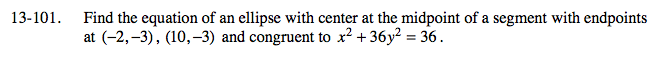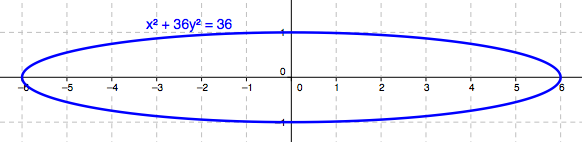### Home > A2C > Chapter 13 > Lesson 13.2.1 > Problem13-101

13-101.Make the given equation equal to 1.

$\frac{x^2}{36}+\frac{y^2}{1}=1$

Find the midpoint of (−2, −3) and (10, −3).

(4, −3)

Write the equation of the congruent ellipse given the information in the hints.